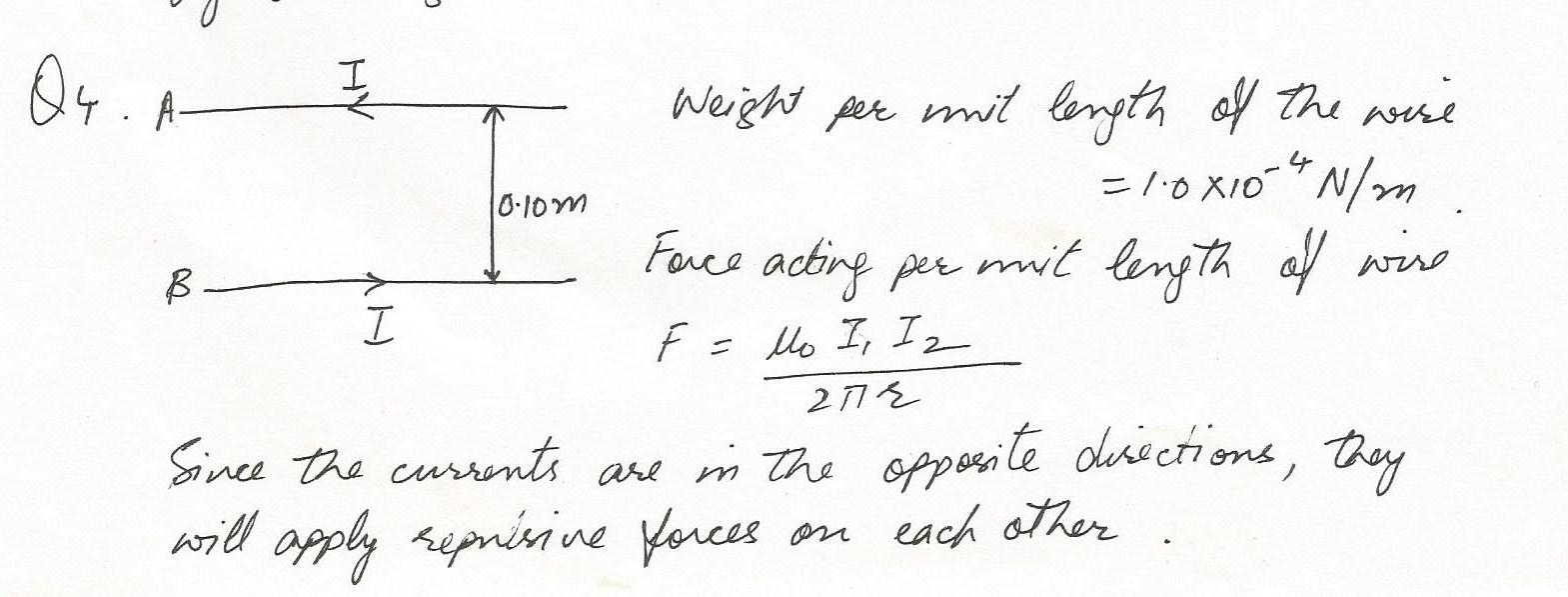8160

Chemistry Organic Chemistry Level: Misc Level

benzonitrile to ethyl benozoate8156

Chemistry Inorganic Chemistry Level: Misc Level

Write the net ionic equation of the reaction between aqueous ammonia,NH4OH and sulfuric acid.8155

Chemistry Inorganic Chemistry Level: Misc Level

A 0.785 M solution of H2SO4 has been prepared as you described in question(1).Thereafter,50 mL of this solution was transferred to another container and diluted to 150 mL to prepare for titration.During titration, it was observed that it took 16.82 mL of the dilute sulfuric acid solution to titrate 25 mL of Na NaOH.What is the molarity of sodium hydroxide?8154

Chemistry Inorganic Chemistry Level: Misc Level

You are asked to preoare 500 mL of a 0.785 M solution of H2SO4. Concentrated sulfuric acid has a density of 1.8305 g/mL.

a) How many mL of H2SO4 do you need? b.) Describe how you will prepare the solution.8153

Chemistry Inorganic Chemistry Level: Misc Level

How many grams of the excess reagent are unreacted8152

Chemistry Inorganic Chemistry Level: Misc Level

Identify the limiting reactant8151

Chemistry Inorganic Chemistry Level: Misc Level

How many grams of aluminum sulfate are produced when reacting 15.00 g aluminum with 10.00 g of sulfuric acid8150

Chemistry Inorganic Chemistry Level: Misc Level

Given the equation ;2AI+3H 2SO4 ---> AI2(SO4)3+ 3H28149

Chemistry Inorganic Chemistry Level: Misc Level

How many liters of O2 are required (assume STP) to form 250.0 g of water8148

Chemistry Inorganic Chemistry Level: Misc Level

How many grams of NO can be formed from the reaction of 25.0 grams NH38147

Chemistry Inorganic Chemistry Level: Misc Level

How many moles of O2 are required for the reaction of 5.00 Liters of NH3.8145

Chemistry Inorganic Chemistry Level: Misc Level

Write all mole ratios for the equation; 2C4H6+11O2 ----> 8CO2+ 6HO8144

Chemistry Inorganic Chemistry Level: Misc Level

What is the mass of butane and oxygen (reactants) in the equation; 2C4H6+11O2 ----> 8CO2+ 6HO8143

Chemistry Inorganic Chemistry Level: Misc Level

Verify the conservation of mass law using molar masses of reactants and products for each substance in the equation;

CH4+2O2 ---->CO+ 2H2O8141

Chemistry Inorganic Chemistry Level: Misc Level

Given 3.00 x1020 molecules of CO2 are produced, how many liters of O2 were required to produce this amount?Displaying 1-15 of 6371 results.